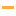Math Games

Play the best free online Math Games! Check out the Top 100 Math games from PrimaryGames.

#81Two Minute Warning: Multiplication Flashcards - By 12Learn multiplication facts and how to multiply by 12 in this online flashcard game. You have 2 minutes to solve as many multiplication problems as you can. Use the keyboard or touchscreen to play and become a times table expert.

#82The Multiplication Game
#83Two Minute Warning: Multiplication Flashcards - By 11Learn multiplication facts and how to multiply by 11 in this online flashcard game. You have 2 minutes to solve as many multiplication problems as you can. Use the keyboard or touchscreen to play and become a times table expert.

#84Click Math: Halloween Addition to 100Practice addition skills with sums up to 100 in this fun puzzle game.

#85Hanukkah CountIt
#86Two Minute Warning: Subtraction Flashcards - HardPractice two-digit subtraction skills in this online flashcard game. You have 2 minutes to solve as many subtraction problems as you can. Use the keyboard or touchscreen to play. Are you looking to practice other math skills? Check out the entire Two...

#87Two Minute Warning: Division Flashcards - By 11Learn division facts and how to divide by 11 in this online flashcard game. You have 2 minutes to solve as many division problems as you can. Use the keyboard or touchscreen to play and become a division table expert.

#88Quick Calculate
#89Math Tiles: St. Patrick's Day Addition and SubtractionPractice addition and subtraction skills in this fun math game. Select from adding, subtracting or both and choose between a range of 10, 20, 100 or 1,000.

#90Comparing NumbersPractice comparing two numbers between 1 and 10 in this fun online math game. The alligators are hungry! They have a huge appetite and always want to eat the bigger number. Practice saying if one number is less than, greater than, or equal to another...

#91Santa CountIt
#92Math Riddles: ChristmasLook at each picture math equation. Use the information from equation to solve for the question mark. What number should replace the question mark?

#93Math Tiles: Easter Addition and SubtractionPractice addition and subtraction skills in this fun puzzle game. Select from adding, subtracting or both and choose between a range of 10, 20 or 100.

#94Valentine Patterns
#95Learn To Count With SpinIt: Christmas EditionPractice counting skills in this fun game. Each question will ask you to turn a certain number of animals. Click on the animals to get them spinning. When the number of spinning animals matches the number in the question, press the green...

#96Math Tiles: Addition 0-20Practice addition skills with sums up to 20 in this fun puzzle game.

#97Winter Patterns
#98Easter Addition Link PuzzlePractice addition skills by matching the correct answer to each question. Complete all of the questions to reveal an Easter picture.

#99Easter Eggs CountIt
#100Thanksgiving CountIt